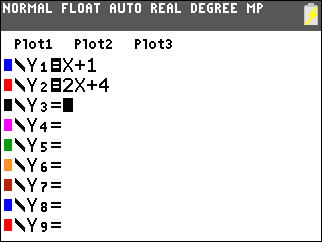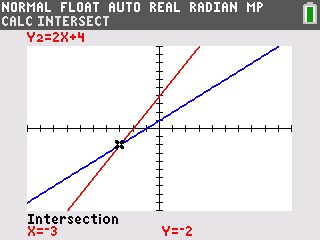# Knowledge Base

## Solution 34471: Finding the Intersection of Two Functions on the TI-84 Plus C Silver Edition Graphing Calculator.

### How can I find the intersection of two functions on the TI-84 Plus C Silver Edition Graphing Calculator?

The intersect operation (selectable from the CALCULATE menu) finds the coordinates of a point at which two or more functions intersect using solve(). The intersection must appear on the display to use intersect.

Refer to the following example in order to calculate the intersection of the graphs of two functions.

Example:

Find the intersection of the following functions:
y = x + 1
y = 2x + 4

Solution:

1) Press [Y=] to display the Y= Editor.
2) Press [X] [+]  [ENTER].
3) Press  [X] [+]  [ENTER].4) Press [GRAPH] to display the graphs of the two functions
5) Press [2ND] [CALC] .
6) When "First curve?" is displayed, press [▲] or [▼] if necessary to move the cursor to the first function.
7) Press [ENTER].
8) When "Second curve?" is displayed, press [▲] or [▼] if necessary to move the cursor to the first function.
9) Press [ENTER].

Please Note:  The calculator requires that a first and second curve be selected in case there are more than two functions being graphed at one time. This is to specify which two functions to find the intersection of in that case.

10) When "Guess?" is displayed, use [◄] and/or [►] to move the cursor to the point that is the guess as to the location of the intersection.
11) Press [ENTER].

Please Note: The calculator requires that a guess be made in case the functions being graphed intersect more than once. This is to specify which intersection of the two selected functions to find in that case.

The cursor is on the solution and the coordinates are displayed, even if CoordOff format is selected. Intersection is displayed in the bottom-left corner. To restore the free-moving cursor, press any of the directional keys.Please see the TI-84 Plus C Silver Edition guidebook for additional information.